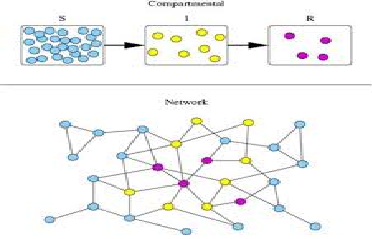# Mathematical Model to Simulate Infectious Disease

Mathematical models allow us to extrapolate from current information about the state and progress of an outbreak, to predict the future and, most importantly, to quantify the uncertainty in these predictions. Many sources of data are used in mathematical modeling, with some forms of model requiring vastly more data than others. However, a good estimation of the number of cases is vitally important. We stretch existing knowledge on model formulation and develop an enhanced mathematical model to study the pattern of spread of infectious diseases. The SIR model formalism was used to compartmentalize the population and the resulting model equations were solved numerically. The disease free equilibrium and endemic equilibrium of the system were established and analyzed for stability. A graph representation of the sub groups is presented and discussed based on the results from simulation.

In many countries of the developed World, infectious diseases of the childhood in particular have been generally conquered. This however, is far from being the case in developing countries, where they are responsible for 45% of all death. Coming down home, the data of infectious disease prepared by the Department of Health Planning and Research, Federal Ministry of Health at our disposal shows the same trend in Rivers State.
A mathematical model is a set of equations, which are the mathematical translation of hypothesis (or assumptions) when interpreting model prediction. It is thus important to bear in mind the underlying assumptions. An assumption, by our definition, is an unverified proposition, tentatively accepted to explain certain facts or to provide a basis for further investigation.
Following analysis, modeling infectious diseases demands that we investigate whether the disease spread could attain a pandemic level or it could be wiped out. The equilibrium analysis helps to achieve this.

Akanksha Shukla